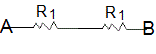# Combination of Resistors — Series and Parallel

Resistors do not occur in isolation. They are almost always part of a larger circuit, and frequently that larger circuit contains many resistors. It is often the case that resistors occur in combinations that repeat. Resistor circuits that combine series and parallel resistors networks together are generally known as Resistor Combination or mixed resistor circuits. The method of calculating the circuits equivalent resistance is the same as that for any individual series or parallel circuit and hopefully we now know that resistors in series carry exactly the same current and that resistors in parallel have exactly the same voltage across them.How to find the equivalent resistance(REQ) for the resistor combination circuit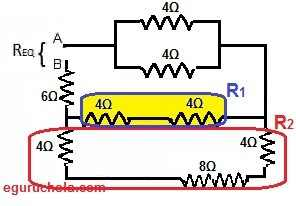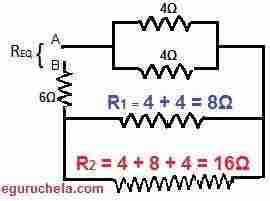(R1 and R1 calculated)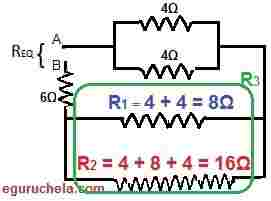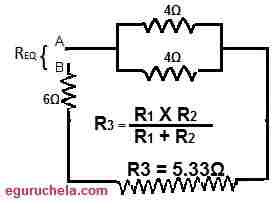(R3 calculated)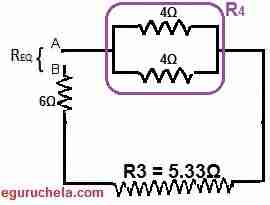(R4 calculated)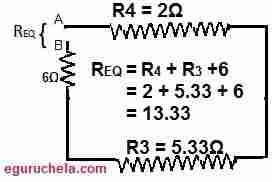### Resistor in Parallel

In the case of parallel connection, the terminals of resistors are connected to the same terminal of the other resistor to form an electronic circuit/ device. Since the resistors connected in parallel therefore common voltage drop in the circuit. The voltage across R1 and R1 is equal. The total resistance in the circuit RTotal:

RTotal = 1/R1 + 1/R2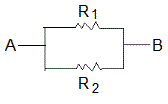### Resistor in Series

In the case of series connection, The resistors are connected is in a single line (sequential array) therefore common current flows in the circuit. The sum of all individual resistor is equal to the total resistance of the circuit RTotal:

RTotal = R1 + R2